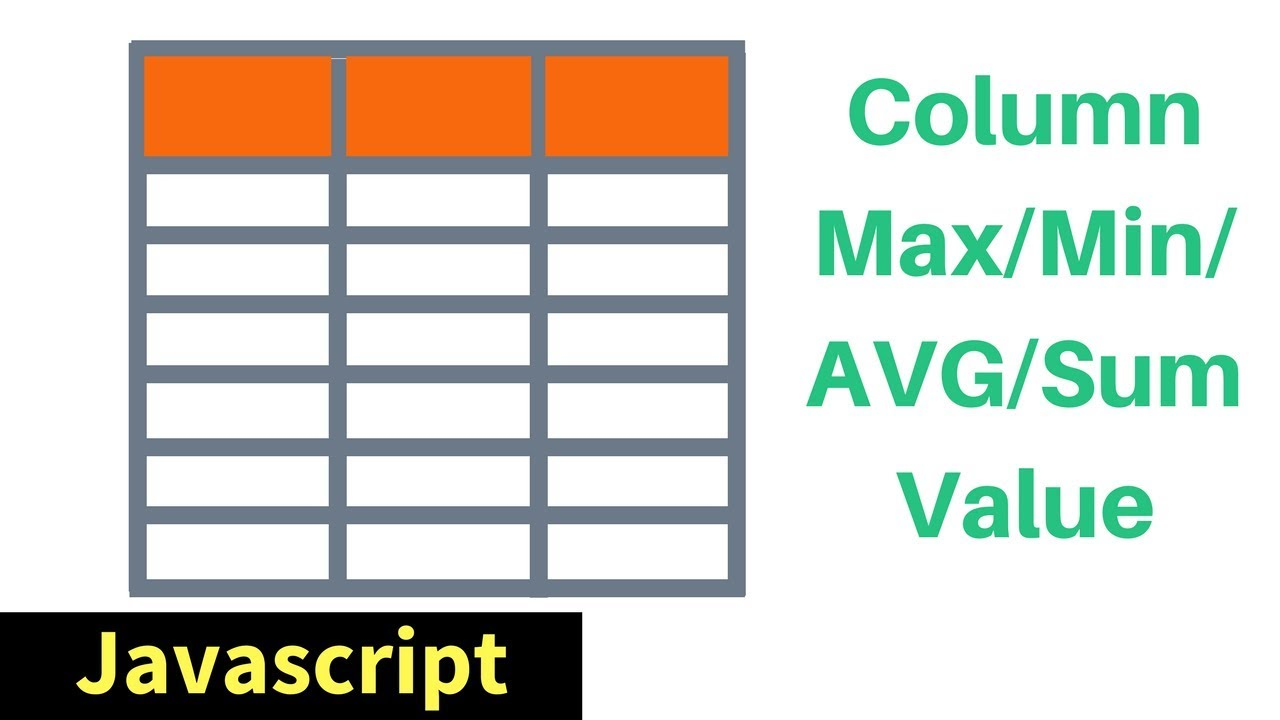# HTML Table Column MAX / MIN / SUM / AVG Values In Javascript [ with source code ]In This Javascript Tutorial we will See How To Find The Maximum, Minimum Value And Calculate The Sum And Average Value Of An HTML Table Column using JS And Netbeans Editor .

In This Javascript Tutorial we will See How To Find The Maximum, Minimum Value And Calculate The Sum And Average Value Of An HTML Table Column using JS And Netbeans Editor .

Project Source Code:

``````
<!DOCTYPE html>

<html>
<title>Javascript HTML Table - Min Max Sum Avg</title>
<meta charset="windows-1252">
<meta name="viewport" content="width=device-width, initial-scale=1.0">
<body>

<table id="table" border="1">
<tr>
<th>First Name</th>
<th>Last Name</th>
<th>Value</th>
</tr>
<tr>
<td>A1</td>
<td>B1</td>
<td>43</td>
</tr>
<tr>
<td>A2</td>
<td>B2</td>
<td>28</td>
</tr>
<tr>
<td>A3</td>
<td>B3</td>
<td>15</td>
</tr>
<tr>
<td>A4</td>
<td>B4</td>
<td>50</td>
</tr>
<tr>
<td>A5</td>
<td>B5</td>
<td>31</td>
</tr>
<tr>
<td>A6</td>
<td>B6</td>
<td>85</td>
</tr>
<tr>
<td>A7</td>
<td>B7</td>
<td>18</td>
</tr>
</table>

<span id="minV"></span><br>
<span id="maxV"></span><br>
<span id="sumV"></span><br>
<span id="avgV"></span>

<script>

var table = document.getElementById("table"), sumVal = 0;

getMin();
getMax();
getSum();
getAvg();

// get min value
function getMin()
{
var minVal, minIndex;
for(var i = 1; i < table.rows.length; i++){
if(i === 1){
minVal = table.rows[i].cells.innerHTML;
minIndex = table.rows[i].rowIndex;
}else if(minVal > table.rows[i].cells.innerHTML){
minVal = table.rows[i].cells.innerHTML;
minIndex = table.rows[i].rowIndex;
}
}
table.rows[minIndex].cells.style.background = "red";
table.rows[minIndex].cells.style.color = "white";
document.getElementById("minV").innerHTML = "Minimum Value = "+minVal;
document.getElementById("minV").style.color = "red";
console.log("Min => "+minVal);
}

// get max value
function getMax()
{
var maxVal, maxIndex;
for(var i = 1; i < table.rows.length; i++){
if(i === 1){
maxVal = table.rows[i].cells.innerHTML;
maxIndex = table.rows[i].rowIndex;
}else if(maxVal < table.rows[i].cells.innerHTML){
maxVal = table.rows[i].cells.innerHTML;
maxIndex = table.rows[i].rowIndex;
}
}
table.rows[maxIndex].cells.style.background = "green";
table.rows[maxIndex].cells.style.color = "white";
document.getElementById("maxV").innerHTML = "Maximum Value = "+maxVal;
document.getElementById("maxV").style.color = "green";
console.log("Max => "+maxVal);
}

// caculate total/sum value
function getSum()
{
for(var i = 1; i < table.rows.length; i++){
sumVal = sumVal + parseInt(table.rows[i].cells.innerHTML);
}
document.getElementById("sumV").innerHTML = "Sum Value = "+sumVal;
console.log("Sum => "+sumVal);
}

// caculate avg value
function getAvg()
{
var avg = sumVal / (table.rows.length - 1);// minus the header
document.getElementById("avgV").innerHTML = "Average Value = "+parseInt(avg);
console.log("Avg => "+parseInt(avg));
}

</script>

</body>
</html>
``````

## The essential JavaScript concepts that you should understand

The essential JavaScript concepts that you should understand - For successful developing and to pass a work interview

## Data Types In JavaScript

JavaScript data types are kept easy. While JavaScript data types are mostly similar to other programming languages; some of its data types can be unique. Here, we’ll outline the data types of JavaScript.

## Introduction With Basic JavaScript

Introduction With Basic JavaScript - Unlike most programming languages, the JavaScript language has no concept of input or output. It is designed to run as a scripting language in a host environment, and it is up to the host environment to provide mechanisms for communicating with the outside world.

## JavaScript Memory Management System

The main goal of this article is help to readers to understand that how memory management system performs in JavaScript. I will use a shorthand such as GC which means Garbage Collection. When the browsers use Javascript, they need any memory location to store objects, functions, and all other things. Let’s deep in dive that how things going to work in GC.

## Create a Line Through Effect with JavaScript

In this post we are going to create an amazing line through effect, with help of CSS and lots of JavaScript. So, head over to your terminal and create a folder LineThroughEffect. Create three files -index.html, main.js and styles.css inside it.